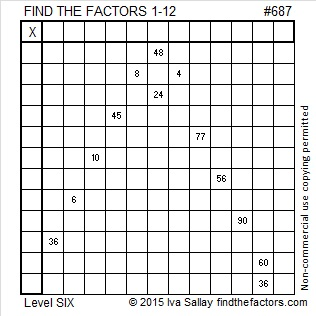# 687 and Level 6

687 is made from three consecutive numbers so it is divisible by 3.

687 is the hypotenuse of Pythagorean triple 180-663-687. What is the greatest common factor of those three numbers?Print the puzzles or type the solution on this excel file: 12 Factors 2015-11-16

—————————————————————————————————

• 687 is a composite number.
• Prime factorization: 687 = 3 x 229
• The exponents in the prime factorization are 1 and 1. Adding one to each and multiplying we get (1 + 1)(1 + 1) = 2 x 2 = 4. Therefore 687 has exactly 4 factors.
• Factors of 687: 1, 3, 229, 687
• Factor pairs: 687 = 1 x 687 or 3 x 229
• 687 has no square factors that allow its square root to be simplified. √687 ≈ 26.21068.—————————————————————————————————Ricardo tweeted the solution for this one: